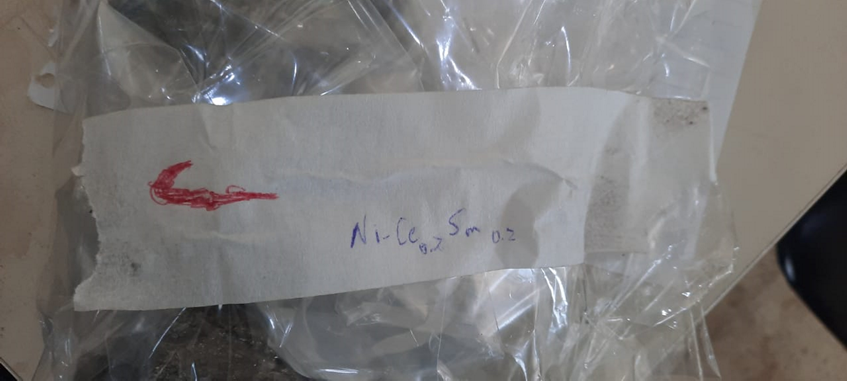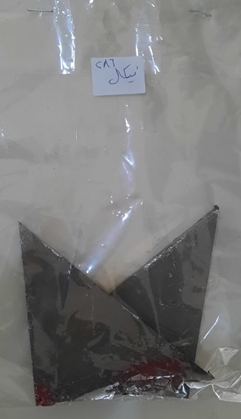# Anode Preparation

Chemical Formula: Ni-Ce0.8Sm0.2

 Metal Melting point Density (g.cm-3) Nickel 1455 oC 8.902 Cerium 795 oC 6.76 Samarium 1072 oC 7.52

Total volume for 100g mass of this alloy = 13.53 cm3

Ƥ = 100/14.2 = 7.391 g/cm3

Volume of the anode (disc shape):

Radius = 6.5 cm; Height = 0.1 cm or more

V = π r2h = π*6.5*0.1 = 13.273 cm3 = 13273 mm3

• If h = 1 mm → V = 13273 mm3 = 13.273 cm3

M1 = Ƥ V = 7.391*13.273 = 98.100743 g

MNi = 286 g

MCe = 510 g

MSm = 147 g

MTotal = 286+510+147 = 943 g

 Metal Mass (g) %W No of moles Xi Nickel 286 30.33 4.873 0.5134 Cerium 510 54.08 3.640 0.3835 Samarium 147 15.59 0.978 0.1030 Alloy 943 100 9.491 1